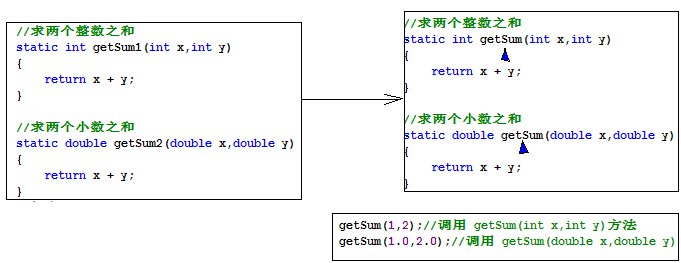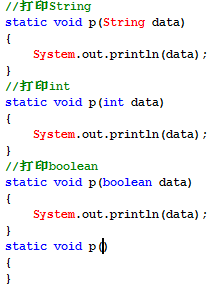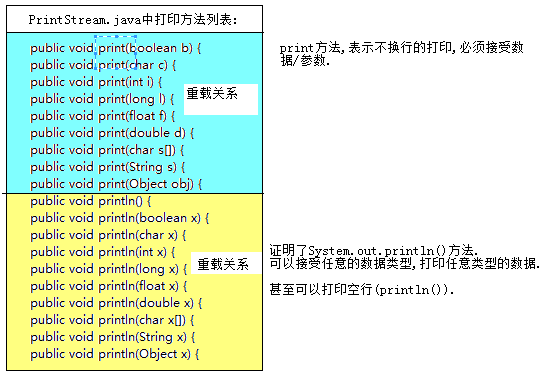# Java-方法的重载设计

```	//求两个数之和
static int getSum1(int x,int y) {
return x + y;
}
static double getSum2(double x,double y) {
return x +y;
}```

```	//打印String
static void pString(String data) {
System.out.println(data);
}
//打印int
static void pInt(int data) {
System.out.println(data);
}
//打印boolean
static void pBoolean(boolean data) {
System.out.println(data);
}```void doWork(int a,char b,boolean c){}

a. void doWork(char b, int a, boolean c){} //YES

b. int doWork(boolean a,char c ,int b){} //YES

c. void doWork(int a,char b,double c){} //YES

d. void doWork(int x,char y,boolean z){} //编译报错

e. int doWork(int x,double y){} //YES

f. int doWork(int x, char y,boolean z){} //编译报错

THE END